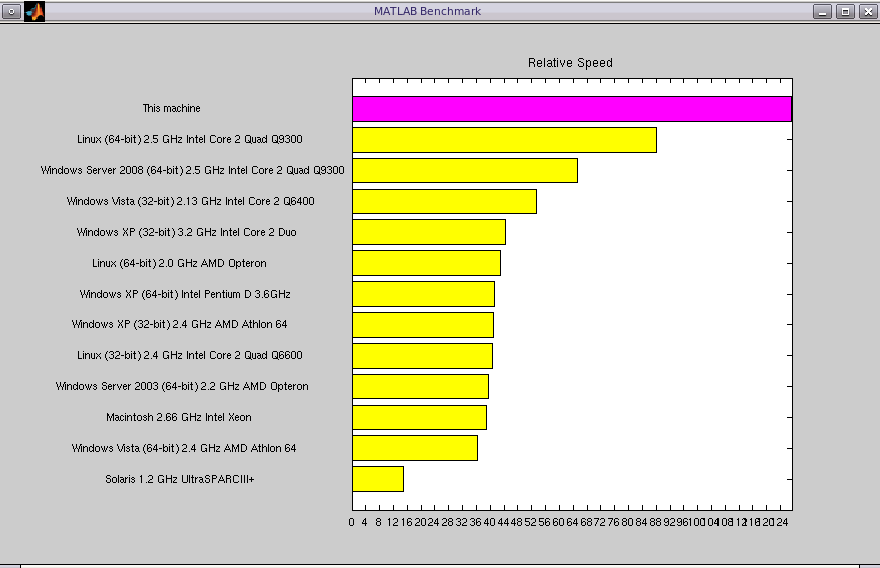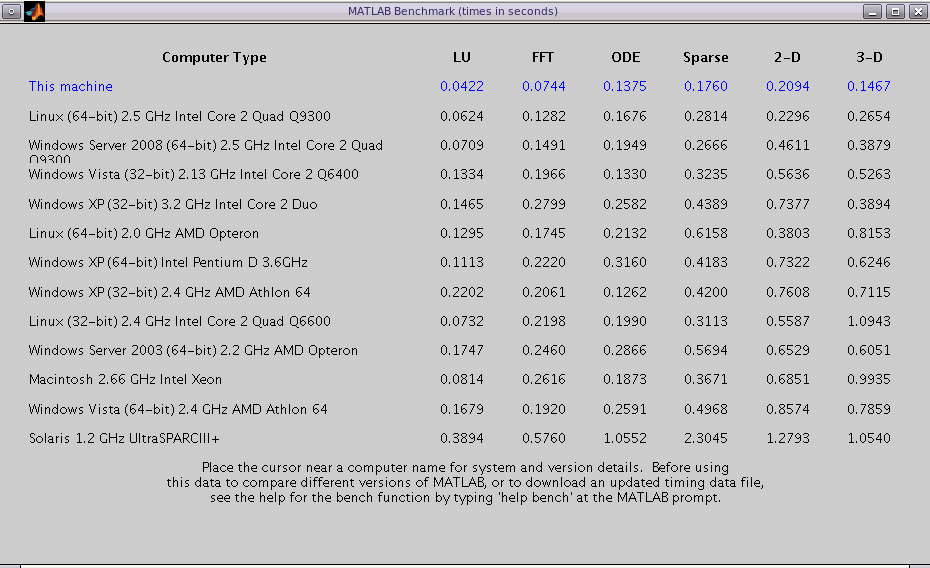# Matlab `bench` Results (June 9, 2009)

The Matlab (7.8.0.347) `bench` function was run on an HP Z800 with
• dual Intel NMH 3.20 GHZ,
• 6 x 2 GB DDR3 1333,
• NVidia FX4800, and
• 4 x 146 GB SAS drives,
listed as   `This machine`  in the following results. Thanks go to HP for the donation of this zippy machine to the Department of Computer Science, Colorado State University!# Execution speed of simple matrix operations in various languages

I have been curious about relative execution speeds for simple matrix calculations performed in Matlab, R, Haskell, and C, to help decide which language to use for my matrix-based machine learning experiments. I have mostly used Matlab and R, but believe Haskell may have advantages in the ease of parallel implementations and the generation of optimized, compiled code.

So, here are some execution times for calculating Z = M * M, followed by Z = Z * M repeated for 49 times, where M is a square matrix. The size, n, of M varies from 5 to 3,125. The implementations in all languages are linked with atlas, except for Matlab. All code is available in this tar file.

Here are the results. Execution times are in milliseconds or seconds for size, n, of matrix from 25 to 3,125. Thanks to Elliot Forney for help with linking with atlas and for writing the C/cublas version.

Matlab R Haskell Python (numpy) C (atlas) C/cublas
HP Z800 n = 25 1.1 ms 2.0 ms 1.3 ms 1.2 ms, 0.95 with reduce 1.0 ms 2.2 ms
n = 125 30.1 ms 63 ms 47 ms 59.8 ms, 16.7 with reduce 42 ms 6.9 ms
n = 625 0.77 s 1.77 s 1.40 s 1.62 s, 0.50 with reduce 1.37 s 0.38 s
n = 3125 76 s 100 s 89 s 167 s, 45.1 with reduce 91 s 43 s

Machines:

• Z800, dual Intel NMH, 8 x 3.20 GHZ, 6 x 2 GB DDR3 1333, NVidia FX4800, 4 x 146 GB SAS drives,